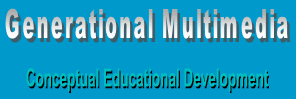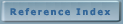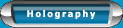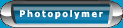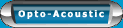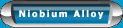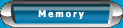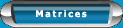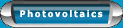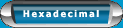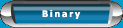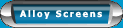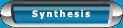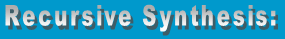Aarnold Hohti, University of Helsinki
 The rigorous articulation of experience dictates that experience is bounded by its forms; that is to say, each element of experience is a form. Particular forms lie in relational fields and are to be considered a possibility or mode of forms to be connected to a given one.  Recursive synthesis, simply described, consists in replacing the many with one, an element to be used in further syntheses.  The synthesized element is related to the replaced ones through the very relation of collecting (synthesis). It both ‘hides’ and ‘opens up into’ the collected elements. The totality therefore is the subset in sum of all parts that comprise the one. With multiple generations, there comes forth the notion of the finite state.  In recursion, this notion of things being finite yields to a transitive nature. The problem to model is the exact universe of subsets that do not exceed the range of any conceivable dimensional assessment. It turns out that the synthetic ‘form’ of the totalities is found in their topological ‘structure’. The concrete forms of mathematical experience are anything that can be given a finite alphabet and finite rules of generation; thus, we have a generative relation or generational construct. Each part of mathematics has its own productive rules for the forms, and its internal relation which make the forms into a relational field.  Thus, the forms in a propositional calculus are its sentences, generated in the standard manner from the logical connectives and propositional symbols. Any boundary that exists is always between an interior and exterior. This is the natural point of contact with topology and modal logic.  The axioms for topology are defined for ‘parts’ of a topological ‘space’.  We consider chains of the form (f,R) consisting of a form ‘f’ and a relation ‘R’ connecting ‘f’ to other forms. Sets are replaced by concepts represented by forms in the given relational system. The radical standpoint of formal experience should be contrasted with that of epistemology, which considers objects of knowledge, not those of experience. The concept of ‘set’ is changed when we move from finite to infinite collections. The decisive step in pure epistemology is that no algorithm exists to create what can not be created. Standard mathematical practice requires that there be a ‘power object’. The crucial question in set theory hinges on the power axiom; that is, do we make the power object a set- especially in light of the fact that set theory requires mathematical objects such as the set of all Real numbers to be represented as sets. Axiomatic set theory cannot provide a full account of the set of real numbers. There exists the possibility of treating the object as the totality, in unity, different from the mere algorithm that created it. This is where set theory fails as a foundation for mathematics as a consequence of the metaphysical layering it has transitively extended across finite boundaries to the formalism of mathematics. This is evidenced in the calculation of a radical in irrational form to a value that can not be recursively synthesized to its original form. The reason for this is very simple. The finite structure of logical space does not solve the recursively synthetic problem of movement beyond the limits of reason defined by boundaries. It must therefore be stipulated that the collecting process of synthesis must be grounded on a simple basis which reduces the general field of such problems. Uniformizaton is essential for mathematics of recursive synthesis. In order to make collecting and computing effective, a uniform scale of recursive levels (powers of 10) was introduced.  Products, or conversely division, are uniform reformulations of syntheses, and uniform scales of products (powers) make a uniform recursive synthesis possible. Recursive synthesis is made possible by the condition that every result of finite collecting is again an element of the domain. Uniformization consists in the finding the units which provide the common ground of the things considered. The introduction of coordinates then allows for a recursive hierarchy of forms transformed into a homogenous field of calculus in which individual operations are simply ‘coordinate-wise arithmetic operations’. Descartes was very much a part of the merging of coordinate geometry with arithmetic computation. He understood that in order to make the movement of thought ‘continuous and nowhere interrupted’ the parts must be arranged in series with simple relations connected clearly by replacing real values with truth values. Boolean operators present the dilemma of propositional truth as analyzed into the truth distribution 1,0,1,1  over (0,0),(1,0),(0,1),(1,1). Aside from the analysis there is the truth that it is any combination of (1,0) then (0,1) that have the highest probability of propagation. Still classical logic was replaced by binary logic. Constructivism perseveres in the talk of sets and dismisses the infinite combination considerations as considered by Hilbert. The purpose of this confirmation is to reaffirm that the constructivism is the core of human experience and the rational ground for mathematical consideration.